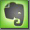Optics

Fujinon Focal Length / Lens Calculator

Focal Length / Focal Distance

Parallel light rays will be focused at a point called the focal point or principal focal point. The distance from the center of the lens to this point is the principal focal length of the lens (for a thin double convex lens). The principal focal length is abbreviated as f.

The focal length is the distance from the optical center of the lens to the image (the film or digital sensor) if focused to an object at infinite distance.

If the image should be focused on something closer than at infinite distance the lens must be moved away from the image, closer to the object. The maximum amount of light a lens can transmit depends on the diameter of the lens and the focal length. The maximum aperture is the focal length divided by the diameter of the lens.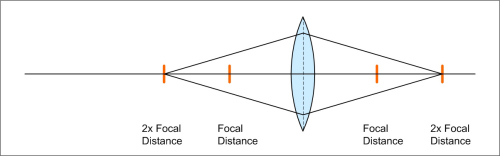from double focal point to double focal point (no image possible)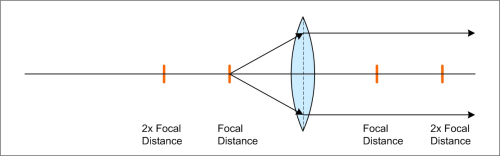from focal point - parallel light rays (no image possible)parallel light rays - into focal point / burning glass effect (no image possible)optical image (typically shown only half from center for analysis)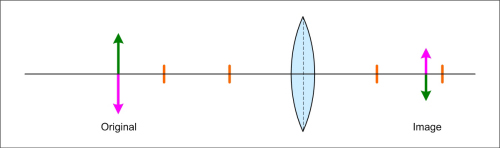full optical image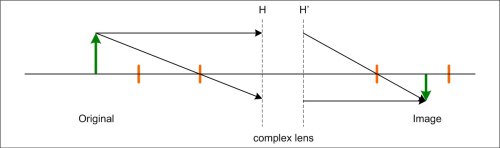real lens system is much more complex, H - H' is used for analysisreal optical lens system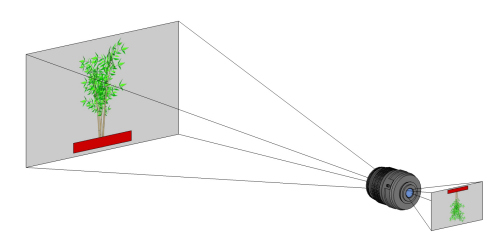object - lens - image

Focal Length and Image Perspective

With photo and video lenses different focal length generates image perspectives with dramatic differences.

 using different focal lengths, used from different positions: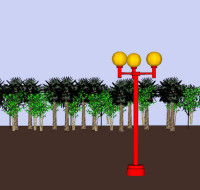long focal length image taken from further distancetelephoto lens medium focal length image taken from medium distancestandard lens short focal length image taken from short distancewide-angle lens using a zoom lens from the same position, changing the zoom factor: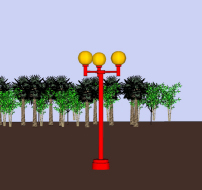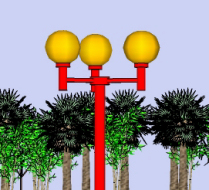zoom lens, further zoom lens, medium zoom lens, nearer